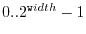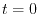Subsections

# Time evolution

libquantum provides two methods for simulating arbitrary quantum systems by solving the time-dependent Schrödinger equation. The first is based on numerical integration, using a fourth-order Runge-Kutta scheme [Press, 1992]. The second is based on exact diagonalization and requires LAPACK to be installed.

## quantum_rk4

 extern void quantum_rk4(quantum_reg *reg, double t, double dt, quantum_reg H(MAX_UNSIGNED, double), int flags);

This function performs a time-step of dt at time t, by calling the function H, which implements the Hamiltonian of the system. H is expected to return a quantum register containing a single row of the Hamiltonian. The expected row is specified by the first parameter to H. The second parameter is the time t, which allows you to solve time-dependent problems. The flags parameter can be set to 1 to prevent deletion of the quantum registers returned by H.

The best way to implement the Hamiltonian is as follows:

1. Determine the number of basis states needed for the current row
2. Create a quantum register with quantum_new_qureg_sparse
3. Fill in the values for the current row
4. Return the quantum register

Note that quantum_rk4 requires reg to be quantum register containing all possible basis states () in ascending order. Here, use the non-sparse quantum_new_qureg_size for initialization of the quantum register. See the example below for further details.

You can even use a limited set of quantum gates concurrently with this function. However, using one of the following gates will result in undefined behavior:

• quantum_cnot
• quantum_toffoli
• quantum_unbounded_toffoli
• quantum_sigma_x
• quantum_sigma_y
• quantum_gate2

## quantum_rk4a

 extern double quantum_rk4a(quantum_reg *reg, double t, double *dt, double epsilon, quantum_reg H(MAX_UNSIGNED, double), int flags);

This function implements the same algorithm as quantum_rk4, but with an adaptive step size, i.e., dt is dynamically adjusted for optimal speed. All parameters correspond to quantum_rk4, except for epsilon, which specifies the desired accuracy. Returns the new step size.

## quantum_diag_time

 extern void quantum_diag_time(float t, quantum_reg *reg0, quantum_reg *regt, quantum_reg *tmp1, quantum_reg *tmp2, quantum_matrix H, float **w);

This function performs a diagonalization of the Hamiltonian given in the matrix H and calculates the state of the quantum system at time t. The initial value atis given by reg0. tmp1 and tmp2 are additional quantum registers, in which data is stored for subsequent calls to quantum_diag_time, so that the time-consuming task of diagonalization has to be performed only once. After the first call to quantum_diag_time, w contains the eigenvalues, and H the eigenvectors of the original matrix, respectively. regt, tmp1, and tmp2 should be initialized prior to the first call, but simply using quantum_new_qureg_size is fine.

In order for this function to work, LAPACK has to be installed and support for it has to be compiled in. Default behavior is to include LAPACK support when available, however this can be changed by using the --without-lapack configure switch.

## Examples

### quantum_rk4

The first example shows an atom in a laser field, undergoing Rabi oscillations. The time-dependent Schrödinger equation is solved by numerical integration using quantum_rk4.

 ``` 1 2 3 4 5 6 7 8 9 10 11 12 13 14 15 16 17 18 19 20 21 22 23 24 25 26 27 28 29 30 31 32 33 34 35 36 37 38 39 40 41 42 ``` ```#include #include #include #define pi 3.14159265358979 const double DE = 1; const double g = 0.1; quantum_reg H(unsigned long long i, double t) { quantum_reg reg = quantum_new_qureg_sparse(2, 1); reg.state = i; reg.amplitude = i*DE; reg.state = 1^i; reg.amplitude = g*sin(DE*t); return reg; } int main() { double T = 2*pi/g; double dt = 0.01; double t; quantum_reg reg; reg = quantum_new_qureg_size(2, 1); reg.amplitude = 1; for(t=0; t

### quantum_diag_time

The second example shows also an atom in a laser field, undergoing Rabi oscillations. However, the Hamiltonian has already been made time-independent by transformation into the rotating frame and performing the rotating wave approximation. The time evolution is then computed by exact diagonalization using quantum_diag_time.

 ``` 1 2 3 4 5 6 7 8 9 10 11 12 13 14 15 16 17 18 19 20 21 22 23 24 25 26 27 28 29 30 31 32 33 ``` ```#include #include #define M(m,x,y) m.t[(x)+(y)*m.cols] #define pi 3.14159265358979 const double g = 0.1; int main() { quantum_matrix h = quantum_new_matrix(2, 2); quantum_reg reg0 = quantum_new_qureg_size(2, 1); quantum_reg regt = quantum_new_qureg_size(1, 1); quantum_reg tmp1 = quantum_new_qureg_size(1, 1); quantum_reg tmp2 = quantum_new_qureg_size(1, 1); float *w; float dt = 0.01; float T = 2*pi/g; float t; M(h, 0, 1) = g; M(h, 1, 0) = g; reg0.amplitude = 1; for(t=0; t